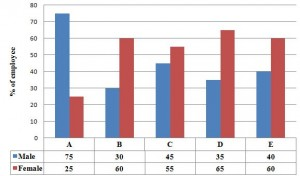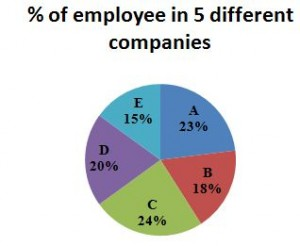Aptitude Questions: Data Interpretation Set 32

Hello Aspirants. Welcome to Online Quantitative Aptitude section in AffairsCloud.com. Here we are creating question sample From Data Intrepretation that is important for all the competitive exams. We have included Some questions that are repeatedly asked in exams !!

I.Study the following graph to answer the given questions

% of Male and Female in 5 different Companies(Total number = 50,000)1. What is the number of female in the company C ?
A.7600
B.5200
C.6600
D.8000
E.None of these
Explanation :
C = 50000*24/100 = 12000
Female = 12000*55/100 = 6600

2. The total number of employees in the company E is approximately what % of the total number of employees in the Company B ?
A.69%
B.93%
C.75%
D.83%
E.None of these
Explanation :
B = 50000*18/100 = 9000
E =50000*15/100 = 7500
% = 7500*100/9000 = 83.33 = 83%

3. What is the difference between number of males working in the company A to number of female working in the company D ?
A.1565
B.4123
C.3240
D.2125
E.None of these
Explanation :
A = 50000*23/100 = 11500
A –Male = 75*11500/100 = 8625
D =50000*20/100 = 10000
D – Female = 65*10000/100 = 6500
Difference = 8625 – 6500 = 2125

4. What is the total number of Males working in the companies B, D and E together ?
A.7600
B.9200
C.10500
D.6700
E.None of these
Explanation :
B = 9000*30/100 =2700
D =10000*35/100 =3500
E =7500*40/100 =3000
Total = 9200

5. What is the ratio between the number of female in Company A to the number of male in Company D ?
A.17:21
B.28:21
C.23:28
D.3:7
E.None of these
Explanation :
A female = 11500*25/100 = 2875
D male = 10000*35/100 = 3500
A:D = 2875 : 3500 =23:28

II.Study the following table to answer the given questions

Number of 5 different newspaper(in thousands) sold in 5 different cities in a month

 City/Newspaper Delhi Mumbai Kolkata Chennai Jaipur The Hindu 630 810 740 420 Hindustan Times 760 650 420 754 560 The times of India 670 563 850 644 Deccan Chronicle 330 643 865 542 The Indian Express 450 312 512 680 346

1. If the number of The Hindu newspaper sold in Kolkata is equal to the difference between the no of The Hindu newspaper sold in the cities Mumbai and Jaipur. Find the average sales of The Hindu news paper in all the 5 cities together in a month ?
A.5,98,000
B.4,59,000
C.4,32,000
D.6,58,000
E.None of these
Explanation :
Kolkata = 810 – 420 = 390
Avg = 630+810+390+740+420 /5 = 598

2. The sales of Deccan Chronicle in Mumbai is what % of that of the Hindustan Times in Chennai.If the no of Deccan Chronicle sold in Mumbai is 100 less than the same newspaper sold in Kolkata?
A.85%
B.72%
C.54%
D.43%
E.None of these
Explanation :
DC M = 543
HT C = 754
% = 543*100/754 = 72.01 = 72%

3. If the Times of India Newspaper sold in Jaipur is equal to the number of The Hindu newspaper sold in Kolkata.Find the average no of newspapers sold in Jaipur in a month.
A.765000
B.543000
C.452000
D.342000
E.None of these
Explanation :
Kolkata The Hindu = Jaipur Times of India = 390
Total = 2258/5 = 451.6 = 452

4. Find the difference between the total no of The Indian Express sold to the total number of Deccan Chronicle sold in a month all the cities together ?
A.623000
B.654000
C.730000
D.432000
E.None of these
Explanation :
The Indian Express = 2300
Deccan Chronicle = 2923
Diff = 2923 – 2300 = 623

5. Find the ratio of number of The Hindu newspaper sold in Delhi to the number of The times of India news paper sold in Kolkata ?
A.12:21
B.44:56
C.63:85
D.3:11
E.None of these